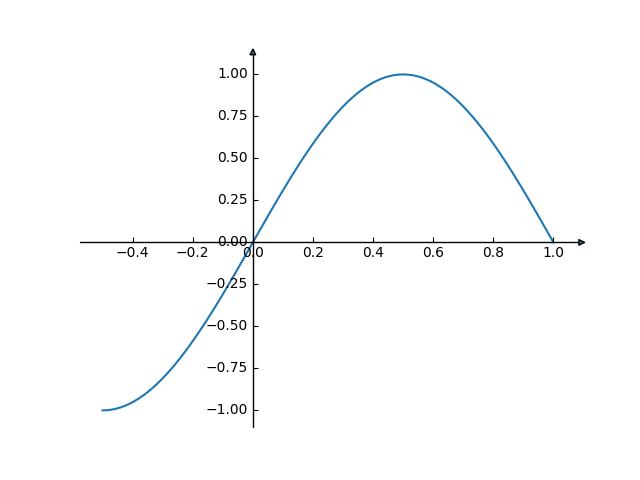# Axis line styles#

This example shows some configurations for axis style.

Note: The mpl_toolkits.axisartist axes classes may be confusing for new users. If the only aim is to obtain arrow heads at the ends of the axes, rather check out the Centered spines with arrows example.from mpl_toolkits.axisartist.axislines import AxesZero
import matplotlib.pyplot as plt
import numpy as np

fig = plt.figure()

for direction in ["xzero", "yzero"]:
# adds arrows at the ends of each axis
ax.axis[direction].set_axisline_style("-|>")

# adds X and Y-axis from the origin
ax.axis[direction].set_visible(True)

for direction in ["left", "right", "bottom", "top"]:
# hides borders
ax.axis[direction].set_visible(False)

x = np.linspace(-0.5, 1., 100)
ax.plot(x, np.sin(x*np.pi))

plt.show()


Keywords: matplotlib code example, codex, python plot, pyplot Gallery generated by Sphinx-Gallery